Components of an electrical circuit or electronic circuit can be connected in many different ways. The two simplest of these are called series and parallel and occur frequently. Components connected in series are connected along a single path, so the same current flows through all of the components. Components connected in parallel are connected along multiple paths, so the same voltage is applied to each component.

A circuit composed solely of components connected in series is known as a series circuit; likewise, one connected completely in parallel is known as a parallel circuit.

## Comparison chart

Parallel Circuit versus Series Circuit comparison chart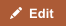Parallel CircuitSeries Circuit
Introduction A circuit composed of components connected completely in parallel. A circuit composed solely of components connected in series.
Wire Comparatively more wire is needed to make it. Comparatively less wire is needed to make it.
Current The current of the circuit from the battery before reaching any component is the sum of all current of the components in a circuit after passing through it. Current of every component of the circuit is same.
Voltage The voltage of every component of the circuit is the same. The voltage of the battery is the sum of all voltage of the components in a circuit.
Functionality Components are functional even if any of the other components is damaged. Components are not functional if any of the components is damaged because it disrupts the flow of current.

## Understanding Series and Parallel Circuits

In a series circuit, the current through each of the components is the same, and the voltage across the circuit is the sum of the voltages across each component. In a parallel circuit, the voltage across each of the components is the same, and the total current is the sum of the currents through each component.

The YouTube video below offers a good explanation of series and parallel circuits, and how the arrangement affects the amount of current flowing through the circuits in accordance with Ohm's Law (even though the thumbnail gives the impression that the video may be broken, it still works):

## Voltage

In a series circuit the voltage is addition of all the voltage elements.

V = V1 + V2 + ... + Vn

In a parallel circuit the voltage is the same for all elements.

V = V1 = V2 = ... = Vn

## Current

In a series circuit, the current is the same for all of the elements.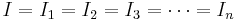$I = I_1 = I_2 = I_3 = \cdots = I_n$

In a parallel circuit, the current in each individual resistor is calculated via Ohm's law.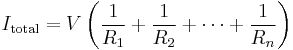$I_\mathrm{total} = V\left(\frac{1}{R_1} + \frac{1}{R_2} + \cdots + \frac{1}{R_n}\right)$.

## Resistors

### Resistance and conductance in series circuits

Total resistance in a series circuit is simply the sum of the resistances of individual resistors.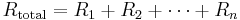$R_\text{total} = R_1 + R_2 + \cdots + R_n$

Conductance is the inverse of resistance. So total conductance of a series circuit is calculated via this equation: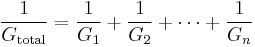$\frac{1}{G_\mathrm{total}} = \frac{1}{G_1} + \frac{1}{G_2} + \cdots + \frac{1}{G_n}$.

### Resistance and conductance in parallel circuits

Total resistance in a parallel circuit is calculated like conductance in a series circuit: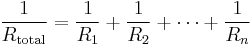$\frac{1}{R_\mathrm{total}} = \frac{1}{R_1} + \frac{1}{R_2} + \cdots + \frac{1}{R_n}$.

Conductance in a parallel circuit is simply the sum of conductance for individual elements:n: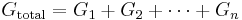$G_\mathrm{total} = G_1 + G_2 + \cdots + G_n$.

## Switches

Two or more switches in series make a logical AND operation. The circuit carries current only if all switches are closed (On). But in a parallel circuit, two or more switches make a logical OR gate. Current flows as long as any one of the switches is closed.